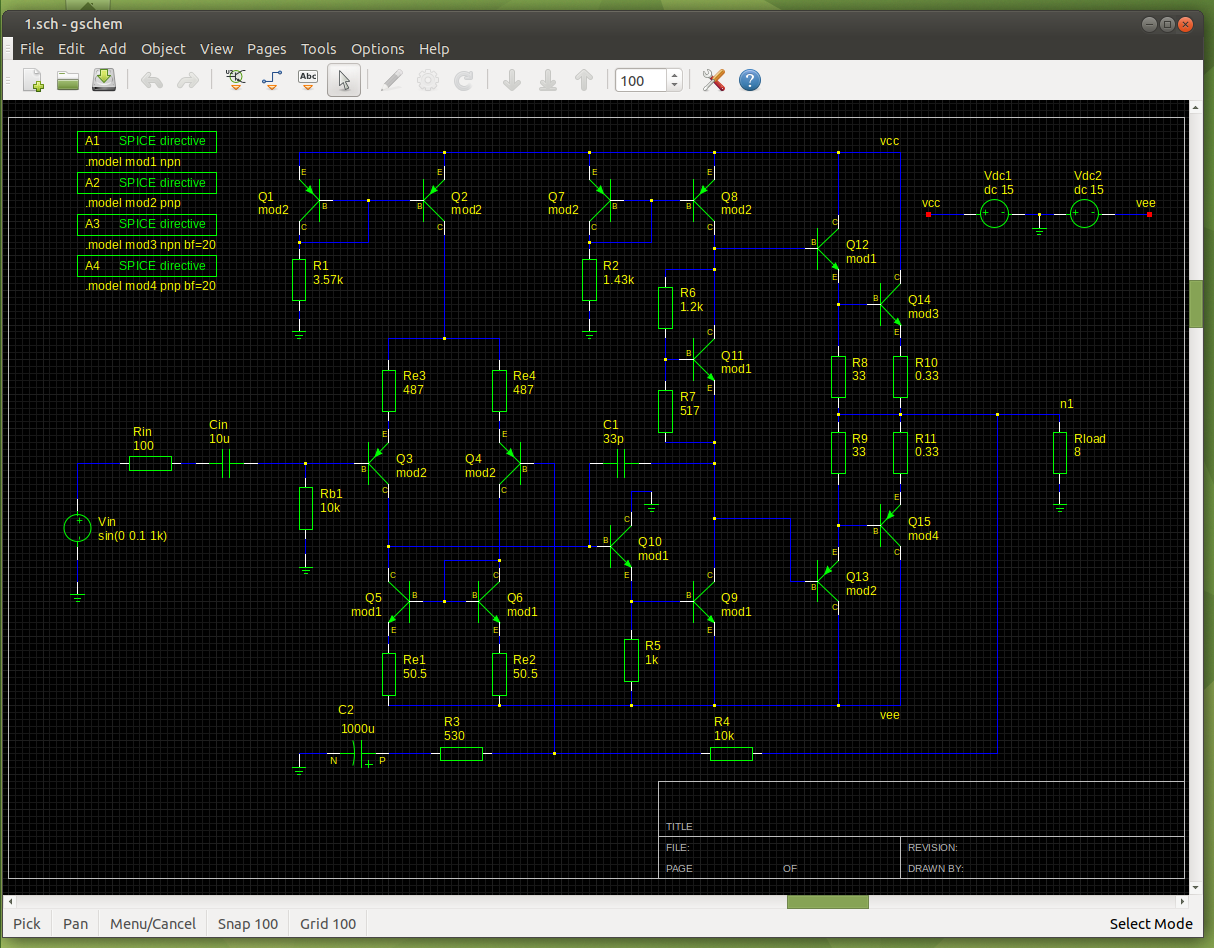# The Output StageThe output stage in this example is a Class AB complementary Darlington configuration, consisting of Emitter Followers Q12 Q13 (drivers) followed with output devices Q14 Q15. The emitter resistors R8 and R9 set the idle current of the drivers at about 21mA (0.7/33 = 0.021). The output stage emitter resistors R10 and R11 provide thermal bias stability and also contribute to controlling crossover distortion. The output stage provides a voltage gain of slightly less than unity, but its main purpose is to buffer the output of the VAS with a large current gain. If we assume the drivers have betas of 100 and the output transistors have betas of 20, then the combined current gain is 2000. When driving an 8Ω load for example, the load impedance seen by the VAS looking into the output stage will be about 16,000Ω.

On positive half cycles of the signal, transistors Q12 and Q14 conduct current and transport the signal to the output node by sourcing current to the load. On negative half cycles, transistors Q13 and Q15 conduct current and transport the signal to the output node by sinking current from the load.

For small signals, if the output stage is biased at 100mA, then the re of each output transistor is about 0.26Ω (0.026/0.1). The summed resistance for each side is then 0.26 + 0.33 = 0.59Ω. As the output halves are in parallel, this results in an output impedance of about 0.3Ω. "If the load impedance is 8Ω, then the voltage gain of the the output stage will be 8/(8 + 0.3) = 0.96".

At high current, re becomes very small, so Zout(large signal) ~= Re (at larger signal swings, only one half of the output stage is active). If Re is chosen so that Re = re(idle) then Zout(large signal) ~= Zout(small signal) ~= Re, and crossover distortion is minimised by making the large signal and small signal output stage gains approximately equal.

"The idle bias current of the output stage plays a critical role in controlling crossover distortion. It is important that the right amount of bias current flows through the output stage, from top to bottom, when the output is not delivering any current to the load." The two driver and two output transistors require at least four Vbe drops from the base of Q12 to the base of Q13 to begin to turn on. The optimum Class AB idle bias current is to provide a voltage drop of approximately 26mV across each of the output resistors:

re = Vt/Ic = 26mV/Ic
Then Ic = 26mV/re = 26mV/Re
Vre = Ic * Re = 26mV

With 0.33Ω emitter resistors, this corresponds to about 79mA. Note that additional resistance results from physical base resistance and emitter resistances inside the transistor, acting like an extension of the external emitter resistor Re. "This means that the optimum voltage drop across the external emitter resistor will be somewhat less than 26mV".

The bias voltage is developed across the bias spreader, which is a Vbe multiplier built around Q11. In practice, R7 is made adjustable to set the required bias current. "The temperature coefficient of the voltage produced by the Vbe multiplier should match approximately that of the base-emitter junction voltages of the output and driver transistors". "The output transistors will usually heat up the most", and as they are mounted on a heat sink, Q11 should also be mounted on the heat sink so that it is exposed to the same approximate temperature.

From Designing Audio Power Amplifiers, Bob Cordell, ISBN: 978-0-07-164025-1

On a final note for this tutorial:
"...with no bias each output device is operating for slightly less than half the time, and the question arises as to whether it would be more accurate to call this Class-C and reserve Class-B for that condition of quiescent current which eliminates, or rather minimises, the crossover artifacts." Moving into what is usually called Class-AB, by increasing the quiescent current, does not make things better. This is due to gm-doubling, the voltage gain caused by both devices conducting simultaneously in the centre of the output voltage range, the Class-A region (from the Audio Power Amplifier Design Handbook, Douglas Self, ISBN: 0 7506 56360).